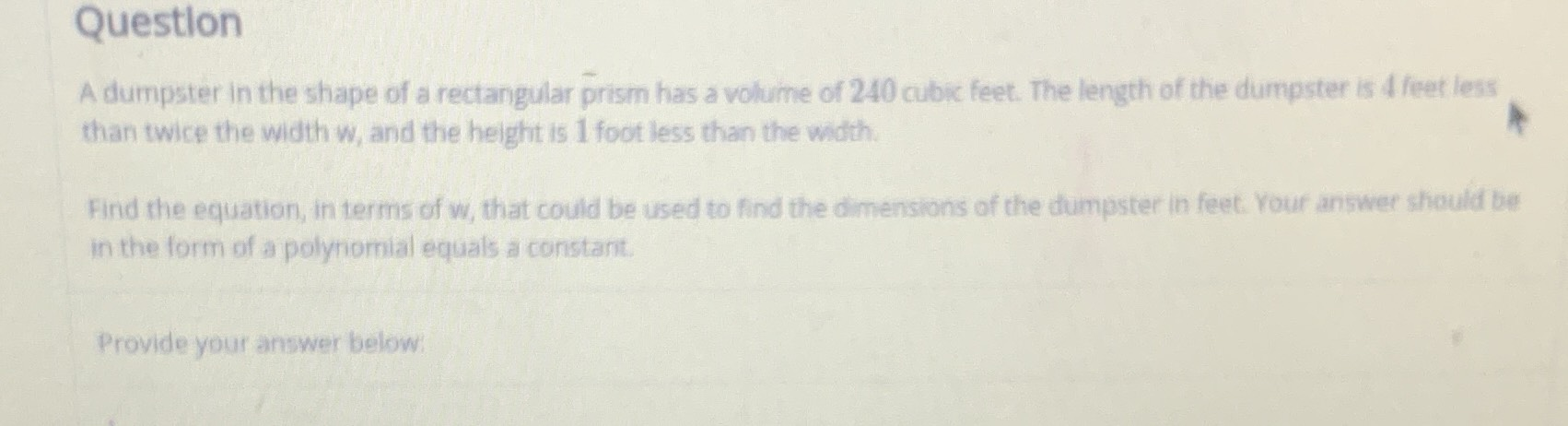### ¿Todavía tienes preguntas de matemáticas?

Pregunte a nuestros tutores expertos
Algebra
PreguntaA dumpster in the shape of a rectangular prism has a volume of $$240$$ cubic feet. The length of the dumpster is $$4$$ feet leas than twice the width w, and the height is $$1$$ foot less than the width.

Find the equation, in terms of w, that could be used to find the dimensions of the dumpster in feet. Your answer should bel in the form of a polynomial equals a constant.